# 工程经济

52
|
2

## 相关模板推荐

•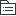## 工程经济 —— 作品大纲

•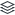资金时间价值
• 概念
•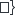时间t、资金大小P、投入回收特点A、周转速度v
• 利息与利率
• 平均利润率为上限
• 供求情况
• 风险
• 通货膨胀
• 时长
• 利息计算
• 单利：F=P*(1+i)*n
• 复利：F=P*(1+i)^n
•• 每季度付息，按季为单利
• 期满还本付息（复利）
• 年末本利和（按一年计算）
•利息——机会成本；利率——息本之比
• 作用：动力、节约、宏观管理
• 名义利率与有效利率
• 名义利率：单利
• 有效利率：复利。值=（1+i)^n-1
• 资金等值
• 现金流量图
• 现值、终值、年金
• F=A (〖(1+i)〗^n-1)/i
•技术方案经济评价
• 方法
• 1. 基本
•确定、不确定（体系指标）
• 确定性分析
• 盈利能力分析
• 静态分析
• 投资收益率
•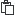优：1、获利能力；2、各种规模投资 缺：1、时间价值
• 总投资收益率
• 资本金净利润率
•自有资金，不包括贷款资金
• 静态回收期
•总投资/年净收益
•年净现金流量均相同直接用公式；不同，看累计净现金流量由正变负，中间值，相似三角形法。 优：补偿速度；缺：未考虑收回后情况
• 动态分析
• 财务内部收益率
•优：1、整个计算周期；2、内部决定性； 缺：1、高次方程多解
• 财务净现值
•已知F求P，P=F/（1+i）^n 优：整个计算周期； 缺：1、基准收益率ic；2、t要相等；3、投资使用效率；4、回收速度
• 基准收益率
•基础：资金成本、机会成本 因素：投资风险、通货膨胀
• 资金成本
• 机会成本
• 投资风险
• 通货膨胀
• 偿债能力分析
•资金来源：1、利润；2、固定资产折旧；3、无形资产及其他资产摊销；4、其他（营业中税金）
• 子主题 7
• 利息备付率
•一般不低于1，我国不低于2
•息税前利润/利息
• 偿债备付率
•一般不低于1，我国不低于1.3
• 借款偿还期
•不预先给定，按最大偿还能力计算
• 资产负债率
• 流动比率
• 速动比率
• 资金来源
• 利润
• 固定资产折旧
• 不属于利润，可用于偿债
• 无形资产摊销
• 类似与折旧
• 其他减免税金
• 生存能力
• 技术方案现金流量表
• 资本金现金流量表
• 投资各方现金流量表
• 财务计划现浇流量表
•要素
• 投资
• 总投资
• 资本金
• 维持运营投资
• 营业成本
• 总成本
• 营业成本
• 营业收入
• 营业收入
• 补贴
• 税金
• 增值税
• 资源税
• 土地增值税
• 附加税
• 不确定性分析
• 盈亏平衡分析
•量本利分析，列方程求解
• 敏感性分析
•指标变化率/因素变化率；S绝对值越大越敏感
• 敏感因素
• 指标
• 因素
• 临界点
•• 2. 性质
•定量、定性
• 3. 时间因素
•静态、动态
• 内容
•盈利能力、偿债能力、生存能力
• 计算期
• 建设期
• 运营期
•利润开始点
• 投产期
• 达产期
•设备租赁与购买
• 租赁
• 融资租赁
•不得终止，设备自有
• 经营租赁
•可取消，设备仅使用权
• 优缺点
•租赁：资金短缺、良好的技术服务、保持资金流动、避免通货膨胀利率波动，能享受税费利益 不足：仅具有使用权、总费用高、长期负债、损坏赔偿
• 租赁费用
• 租赁保证金
• 担保费
• 租金
•给定附加率按附加率法算，否则按年金法。
• 附加率法：R=P*(1/N+i+r)
• 年金法:已知P求A
• 比选方法
• 寿命相同
• 净现值
• 费用现值法
• 寿命不同
• 净年值
• 年成本法
•设备更新
• 磨损与补偿
• 有形
• 外力
• 自然力
• 无形
• 同类设备
• 新设备
• 磨损补偿对应形式
•无形磨损第一种无补偿
• 设备更新
• 策略
•损耗严重、技术落后、经济不合理
• 原则
•客观、不考虑沉默成本、逐年滚动
• 沉默成本
•账面价值-市场价值
• 方法
•自然寿命、技术寿命、经济寿命
• 经济寿命
• 年末残值Ln为常值
•年劣化值
•平均年收益最大：两者相加最小
•强调平均
•价值工程
• 概念：V=F/C
• F：核心、量化、基本功能
• C：生产成本+维护成本（寿命周期成本）
•管理技术、对象价值、时间科研—报废
• 特点
•分析公式：C最低、F功能为核心，F、C为整体研究
• 实施步骤
• 准备
• 分析
• F:干什么
• C；成本多少
• V：价值多少
• =1，最佳
• ＞1可能良好，越大越好
• ＜1，必须改进。一样小看C-F
• 创新
• 方案创造
• 方案评价
• 实施
• 四个落实
• 提高途径
• 双向型
• 改进型
•不花钱
• 节约型
• 投资型
•花钱
• 牺牲型
•新工艺、新技术、新材料经济分析
• 原则
• 技术先进
• 综合效益合理
• 技术分析
• 技术特性
• 技术条件
• 经济分析
• 静态分析
• 增量投资
•给定基准收益率
• 年折算费用
•Z=C+P×Rc
•增加投资比选方法
• 综合总费用
• 研究产量Q
• 动态分析
• 净现值
• 净年值
•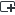融资因素
• 融资前
•4个投资现金流量表、FIRR、P、Pt
•包括动态分析、静态分析
• 投资现金流量表
•技术方案为角度，盈利能力
• 资本现金现金流量表
•技术方案融资（项目法人）为角度
• 投资各方现金流量表
•投资方为角度
• 财务计划现金流量表
•技术方案为角度，生存能力
•四要素
• 营业收入
• 产品收入
• 补贴收入
• 投资
• 建设投资
•实物、工业产权、非专利技术、土地使用权、资源开采权
• 建设期利息
• 流动资金
• 经营成本
•材料、工资、修理、其他为经营成本 外加折旧、摊销、利息为总成本
• 税金
• 融资后
• 动态分析
• 静态分析
•ROE（资本金净利润）、ROI（总投资收益率）